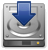# Main Page

## QETLAB: A MATLAB Toolbox for Quantum Entanglement

QETLAB (Quantum Entanglement Theory LABoratory) is a MATLAB toolbox for exploring quantum entanglement theory. While there are many quantum information theory toolboxes that allow the user to perform basic operations such as the partial transposition, new tests are constantly discovered. The goal of QETLAB is to remain up-to-date and contain an ever-growing catalogue of separability criteria, positive maps, and related functions of interest. Furthermore, QETLAB is designed to work well both with full matrices and with large sparse matrices, and makes use of many advanced techniques based on semidefinite programming.Download and Install QETLABStep 1: Download QETLAB (current version: 0.9, last updated: January 12, 2016)Step 2: Unzip the file in your MATLAB scripts directoryStep 3: Download and install CVX 2.1If you need more detailed installation instructions, click here.

## Getting started: some introductory examples

### Pure state entanglement and the Schmidt decomposition

The heart of QETLAB is a suite of functions that make working with quantum entanglement quick and easy. Working with pure state entanglement is a breeze, as demonstrated by the following code snippet, which generates a random pure state (according to uniform spherical measure/Haar measure) and computes its Schmidt decomposition:

>> d = 3; % this example will be in 3 \otimes 3 dimensions
>> phi = RandomStateVector(d^2);
>> [s,a,b] = SchmidtDecomposition(phi)

s =

0.9159
0.3951
0.0709

a =

-0.8062            -0.5411             0.2392
-0.2685 - 0.0526i   0.0305 + 0.2555i  -0.8357 + 0.4006i
0.1580 + 0.5002i  -0.3599 - 0.7152i  -0.2814 + 0.0681i

b =

-0.1401 - 0.3521i   0.0829 - 0.7178i  -0.3997 - 0.4176i
-0.2513 + 0.1492i  -0.3837 + 0.3479i   0.0874 - 0.7992i
0.5520 - 0.6828i  -0.3670 + 0.2737i  -0.1378 - 0.0208i

We could verify that this Schmidt decomposition is correct as follows:

>> phi2 = s(1)*kron(a(:,1),b(:,1)) + s(2)*kron(a(:,2),b(:,2)) + s(3)*kron(a(:,3),b(:,3));
>> norm(phi - phi2)

ans =

5.8378e-016

However, QETLAB has a built-in function that acts as the inverse of the Schmidt decomposition, to make this process easier:

>> phi3 = TensorSum(s,a,b);
>> norm(phi - phi3)

ans =

5.8378e-016

### Mixed state entanglement and detecting bound entanglement

QETLAB can also handle mixed state entanglement without trouble. To demonstrate these features, let's start by creating a bound entangled state and then detecting its entanglement.

One well-known way to create bound entangled states is via unextendible product bases (UPBs). Let's first generate the "Tiles" UPB in $\mathbb{C}^3 \otimes \mathbb{C}^3$ and construct a bound entangled state from it:

>> u = UPB('Tiles') % generate the "Tiles" unextendible product basis

u =

0.7071         0         0         0    0.3333
-0.7071         0         0         0    0.3333
0    0.7071         0         0    0.3333
0         0         0    0.7071    0.3333
0         0         0         0    0.3333
0   -0.7071         0         0    0.3333
0         0         0   -0.7071    0.3333
0         0    0.7071         0    0.3333
0         0   -0.7071         0    0.3333

>> rho = eye(9) - u*u'; % rho is the projection onto the orthogonal complement of the UPB vectors
>> rho = rho/trace(rho); % scale rho to have trace 1

Now rho is a bound entangled state. We can verify this in QETLAB as follows:

>> IsPPT(rho) % this will show that rho has positive partial transpose

ans =

1

>> IsSeparable(rho) % this will show that rho is entangled

Determined to be entangled via the realignment criterion. Reference:
K. Chen and L.-A. Wu. A matrix realignment method for recognizing entanglement. Quantum Inf. Comput., 3:193-202, 2003.

ans =

0

QETLAB can also carry out many related functions, such as finding entanglement witnesses that detect entanglement in a given state, determining whether or not a given operator is an entanglement witness, and deciding whether or not a given set of vectors form an unextendible product basis. Furthermore, there are numerous examples scattered throughout the documentation that make learning how to carry out these operations a breeze.